「啥是佩奇？」

「我用 Mathematica 画给你看！」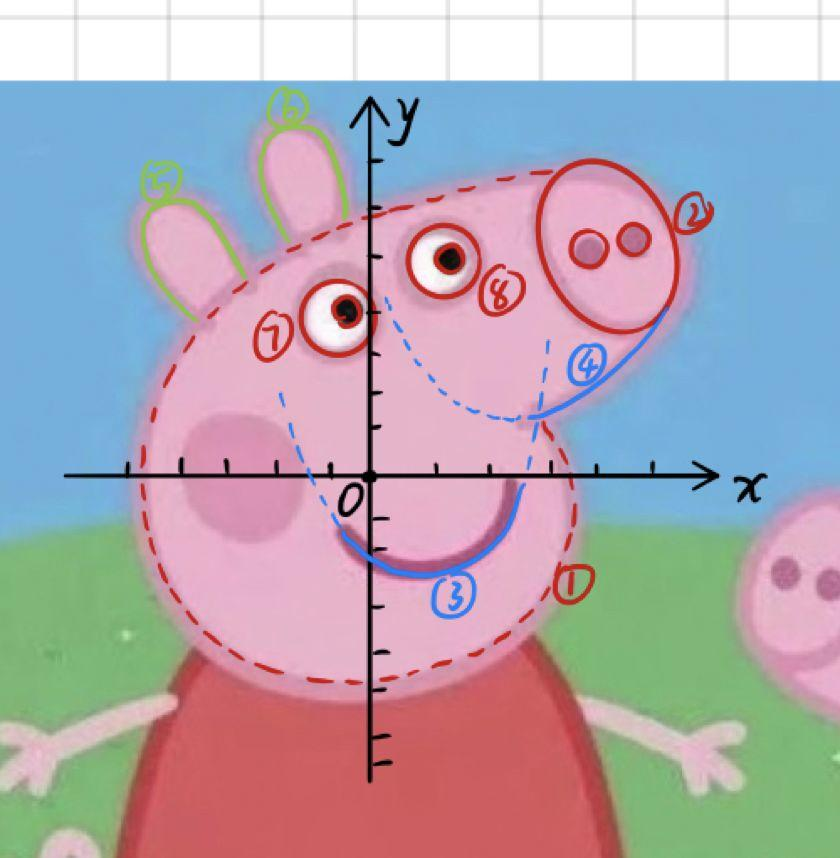Mathematica 是备受科研人员喜爱的编程工具，它神通广大，详细了解请点下面的链接：（视频教程在文末）

1.佩奇的脸：

(1 + t^10){1Cos[[Pi]/6 - 2[Pi] (1/16 + 15/16t)], Sin[[Pi]/6 - 2 [Pi](1/16 + 15/16t)]}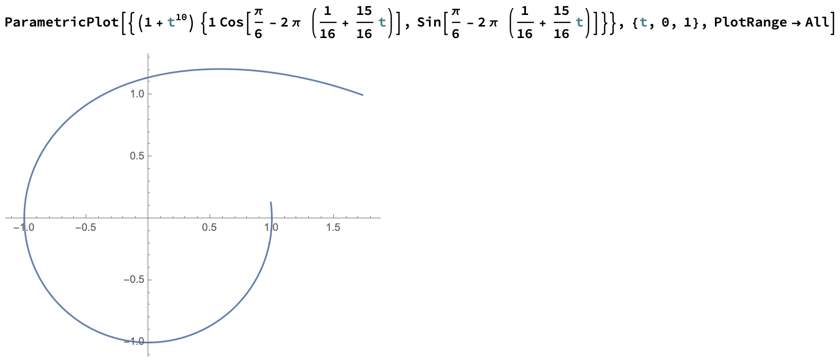2.佩奇的下巴：

{t*4/5+ 1, 1/3t^2+ 1/7}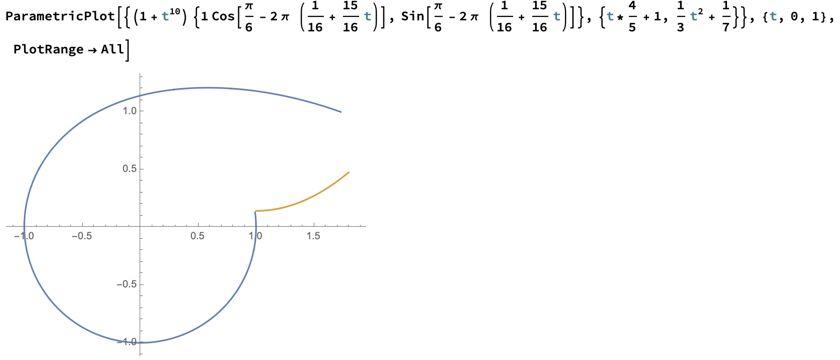3.佩奇的鼻子：

{1/4 Cos[2 [Pi] t] + 8/5, 1/3 Sin[2 [Pi] t] + 7/10}, {1/16 Cos[2 [Pi] t] + 8/5 - 1/8, 1/16 Sin[2 [Pi] t] + 7/10}, {1/16 Cos[2 [Pi] t] + 8/5 + 1/8, 1/16 Sin[2 [Pi] t] + 7/10}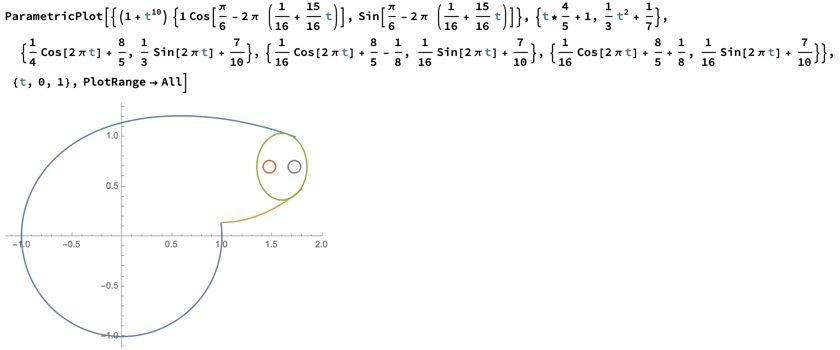4.佩奇的眼睛：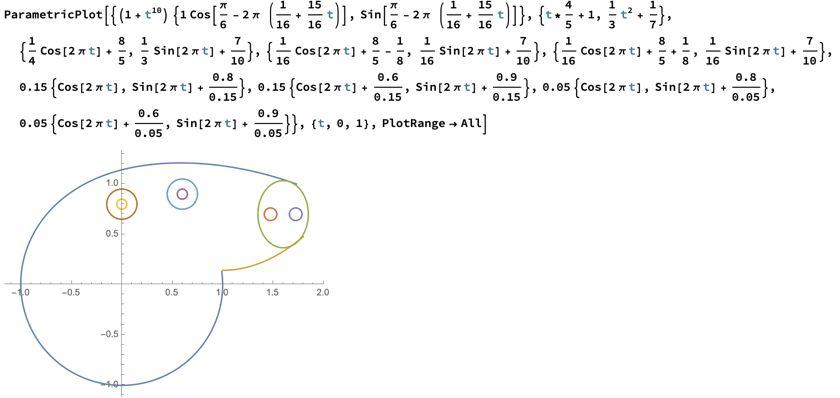5.佩奇的嘴巴：

{t - 1/3, (t - 2/5)^2 - 1/2}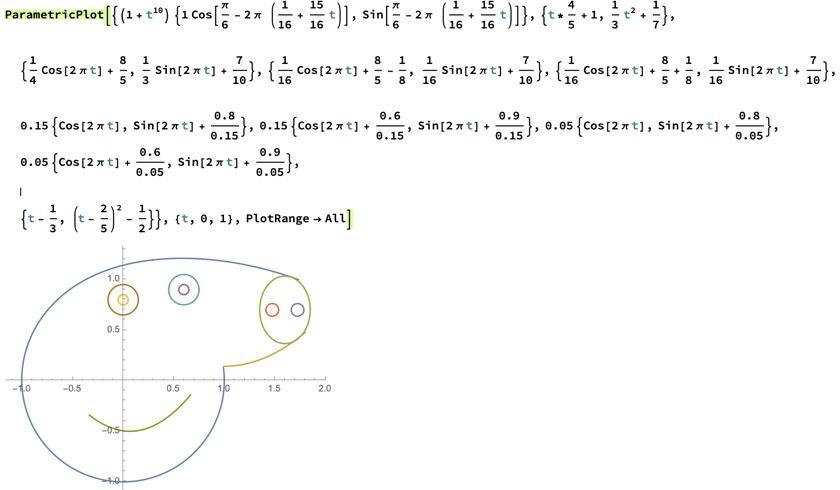6.佩奇的耳朵：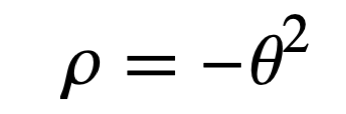{(3/2 - 2 (t - 1/2)^2) Cos[ t/3 + (2 [Pi])/3], (3/2 - 2 (t - 1/2)^2) Sin[ t/3 + (2 [Pi])/3]}, {(3/2 - 2 (t - 1/2)^2) Cos[ t/3 + [Pi]/2], (3/2 - 2 (t - 1/2)^2) Sin[ t/3 + [Pi]/2]}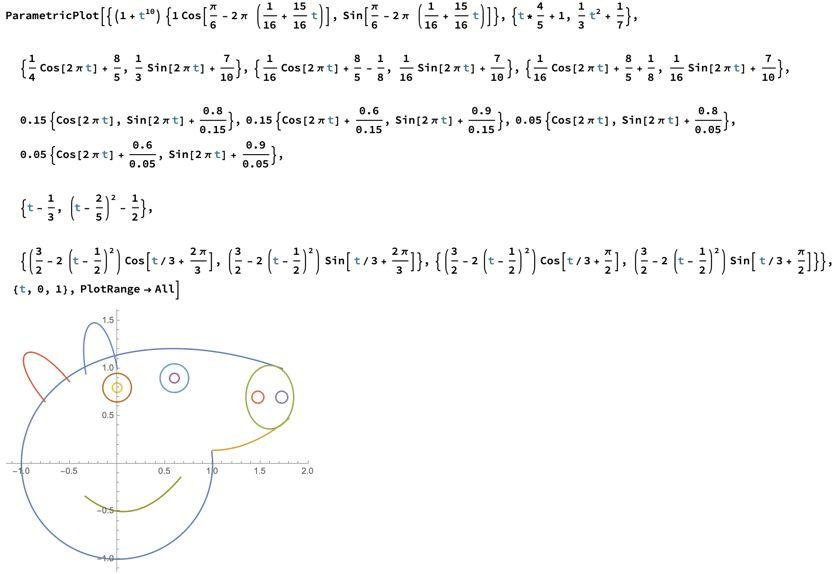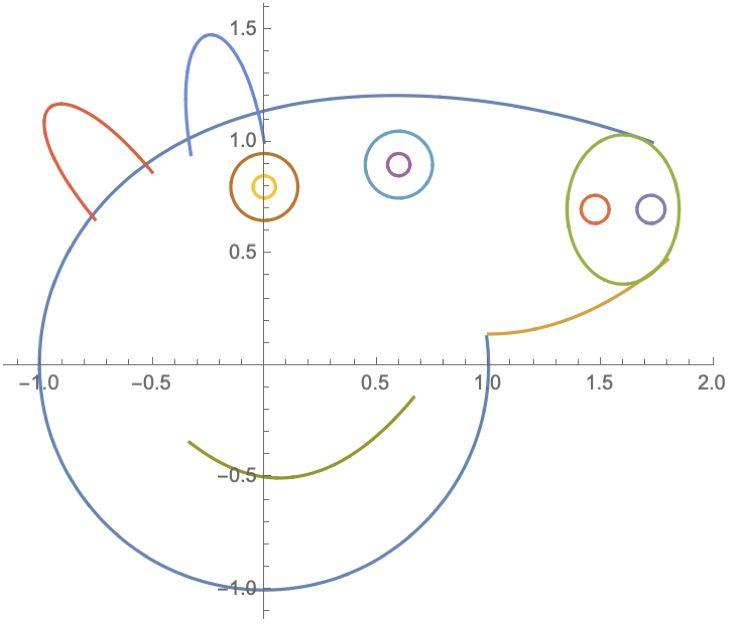Mathematica 版小猪佩奇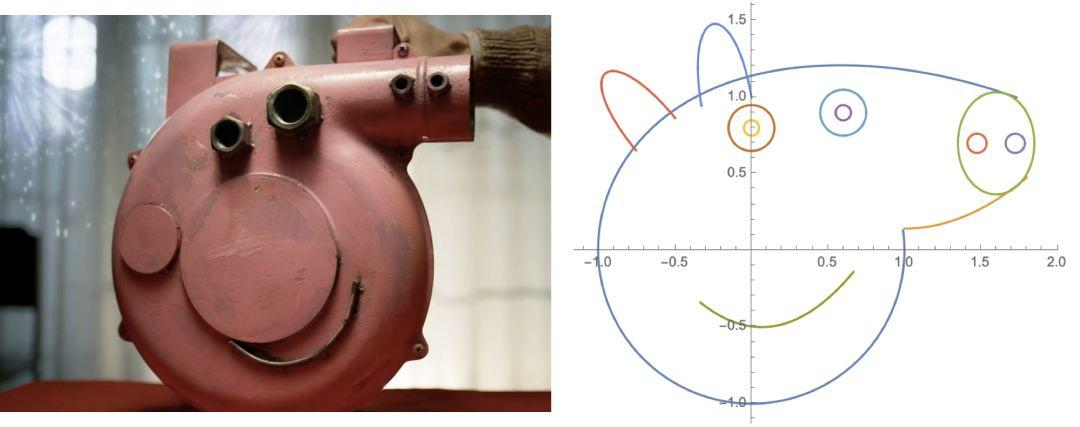ParametricPlot[{(1 + t^10) {1 Cos[[Pi]/6 - 2 [Pi] (1/16 + 15/16 t)], Sin[[Pi]/6 - 2 [Pi] (1/16 + 15/16 t)]}, {t*4/5 + 1, 1/3 t^2 + 1/7}, {1/4 Cos[2 [Pi] t] + 8/5, 1/3 Sin[2 [Pi] t] + 7/10}, {1/16 Cos[2 [Pi] t] + 8/5 - 1/8, 1/16 Sin[2 [Pi] t] + 7/10}, {1/16 Cos[2 [Pi] t] + 8/5 + 1/8, 1/16 Sin[2 [Pi] t] + 7/10}, 0.15 {Cos[2 [Pi] t], Sin[2 [Pi] t] + 0.8/0.15}, 0.15 {Cos[2 [Pi] t] + 0.6/0.15, Sin[2 [Pi] t] + 0.9/0.15}, 0.05 {Cos[2 [Pi] t], Sin[2 [Pi] t] + 0.8/0.05}, 0.05 {Cos[2 [Pi] t] + 0.6/0.05, Sin[2 [Pi] t] + 0.9/0.05}, {t - 1/3, (t - 2/5)^2 - 1/2}, {(3/2 - 2 (t - 1/2)^2) Cos[ t/3 + (2 [Pi])/3], (3/2 - 2 (t - 1/2)^2) Sin[ t/3 + (2 [Pi])/3]}, {(3/2 - 2 (t - 1/2)^2) Cos[ t/3 + [Pi]/2], (3/2 - 2 (t - 1/2)^2) Sin[ t/3 + [Pi]/2]}}, {t, 0, 1}, PlotRange -> All]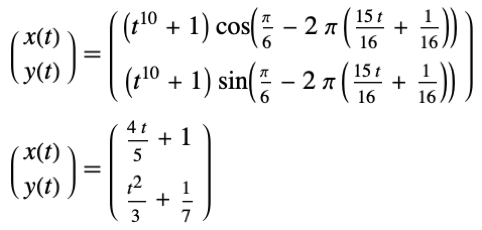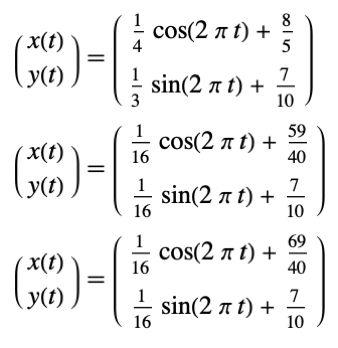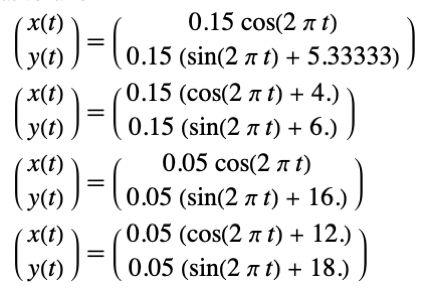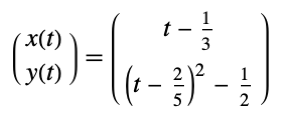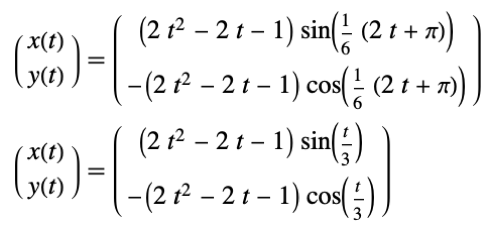《环球科学》杂志和旗下公众号“科研圈”评出了“2018 明星学术公众号”入围榜单，同时也拉开了TOP 10评选的大幕。◆◆◆